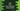# How to read elements of a Java Vector using iterable## How to read elements of a Java Vector using iterable :

The Vector class in Java implements a growable array of objects. Unlike arrays, the vectors can grow or shrink as required. Accessing an element in a vector is same as like arrays. We can use the index position to access it.

In this tutorial, we will learn how to iterate all elements of a vector in Java.

Our program will take all inputs from the user. First, it will ask how many values to add in the vector. Then it will ask the user to enter each value one by one. Finally, it will print out all these values that were entered by the user.

### Java program :

Let’s check the Java program first :

``````import java.util.Iterator;
import java.util.Scanner;
import java.util.Vector;

public class Main {
public static void main(String[] args) {
//1
int count;

//2
Scanner sc = new Scanner(System.in);
Vector vector = new Vector<>();

//3
System.out.println("Enter the total number of elements : ");
count = sc.nextInt();

//4
for (int i = 0; i < count; i++) {
System.out.print("Enter element for position " + (i + 1) + " : ");
}

//5
Iterator iterator = vector.iterator();

//6
System.out.println("You have entered : ");
while (iterator.hasNext()) {
System.out.println(iterator.next());
}
}
}``````

### Explanation :

The commented numbers in the above program denote the step numbers below :

1. Create one integer variable count to store the item count use will add to the vector.
2. Create one Scanner sc to read the user inputs. Also one Vector variable vector. This vector variable can hold only String values.
3. Now, ask the user to enter the total number of elements to add in the vector. Using Scanner sc, read the value and store it in the variable count.
4. Run one for loop to read and add items to the vector. On each iteration of the loop, ask the user to enter a value to enter. Read and add it to the vector variable. For reading the user input string, we are using sc.next() and for adding it to the vector, we are using add() method.
5. Create one Iterator to iterate through the vector. We can get the iterator for a vector using vector.iterator() method.
6. Finally, print out the string values the user has entered. An iterator is used to iterate through the vector elements. hasNext() method will check if any extra elements left to iterate. While this method is returning try, that means we have extra values to scan. next() method will return the next element in the iteration.

### Sample Output :

``````Enter the total number of elements :
4
Enter element for position 1 : hello
Enter element for position 2 : world
Enter element for position 3 : one
Enter element for position 4 : two
You have entered :
hello
world
one
two

Enter the total number of elements :
5
Enter element for position 1 : 1
Enter element for position 2 : 2
Enter element for position 3 : 3
Enter element for position 4 : 4
Enter element for position 5 : 5
You have entered :
1
2
3
4
5``````

### Conclusion :

We have learned how to iterate a vector using an iterator in Java. Try to run the example program we have shown above and drop one comment below if you have any question.